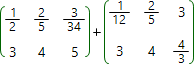Addition operation can only be applied to the matrices of the same size. The result of addition of two matrices is matrix, with elements being equal to the sum of corresponding elements of matrices being added, i.e. if

C = A + B

the elements of matrix C calculated by the formula:

Ci,j = Ai,j + Bi,j

Matrices addition is commutative operation, i.e.

A + B = B + A

A: ×
B: ×A =
B =